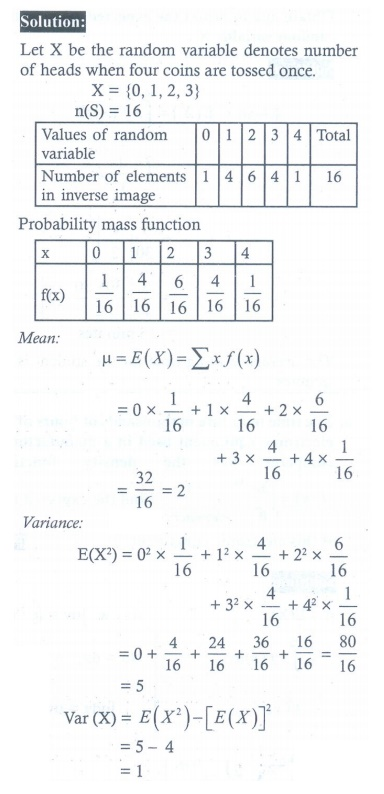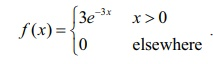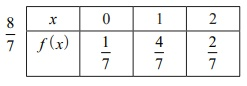Home | | Maths 12th Std | Exercise 11.4: Mathematical Expectation

# Exercise 11.4: Mathematical Expectation

Maths Book back answers and solution for Exercise questions - Mathematics : Probability Distributions: Mathematical Expectation - Exercise Problem Questions with Answer, Solution

EXERCISE 11.4

1. For the random variable X with the given probability mass function as below, find the mean and variance.2. Two balls are drawn in succession without replacement from an urn containing four red balls and three black balls. Let X be the possible outcomes drawing red balls. Find the probability mass function and mean for X.3. If μ and σ 2 are the mean and variance of the discrete random variable X , and E ( X + 3) = 10 and E ( X + 3)2 = 116 , find μ and σ 2.4. Four fair coins are tossed once. Find the probability mass function, mean and variance for number of heads occurred.5. A commuter train arrives punctually at a station every half hour. Each morning, a student leaves his house to the train station. Let X denote the amount of time, in minutes, that the student waits for the train from the time he reaches the train station. It is known that the pdf of isObtain and interpret the expected value of the random variable X .6. The time to failure in thousands of hours of an electronic equipment used in a manufactured computer has the density functionFind the expected life of this electronic equipment.7. The probability density function of the random variable X is given byfind the mean and variance of X .8. A lottery with 600 tickets gives one prize of 200, four prizes of 100, and six prizes of ₹ 50. If the ticket costs is ₹ 2, find the expected winning amount of a ticket.(1) (i) 2.3 , 2.81   (ii) 1.67 , 0.56   (iii) 5/3 , 1/18     (iv) 2,4

(2)(3)(5) 15 minutes    (6) 1/3   (7) 1/2 , 1/8     (8) Loss ₹. 0.50

Tags : Problem Questions with Answer, Solution , 12th Maths : UNIT 11 : Probability Distributions
Study Material, Lecturing Notes, Assignment, Reference, Wiki description explanation, brief detail
12th Maths : UNIT 11 : Probability Distributions : Exercise 11.4: Mathematical Expectation | Problem Questions with Answer, Solution